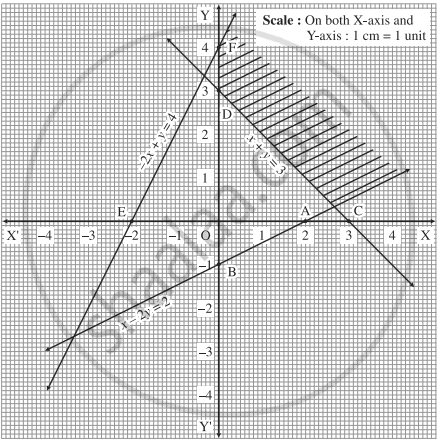Advertisement Remove all ads

# Find the feasible solution of the following inequations: x - 2y ≤ 2, x + y ≥ 3, - 2x + y ≤ 4, x ≥ 0, y ≥ 0 - Mathematics and Statistics

Graph
Sum

Find the feasible solution of the following inequations:

x - 2y ≤ 2, x + y ≥ 3, - 2x + y ≤ 4, x ≥ 0, y ≥ 0

Advertisement Remove all ads

#### Solution

First we draw the lines AB, CD and EF whose equations are x - 2y = 2, x + y = 3 and - 2x + y = 4 respectively.

 Line Equation Points on the X-axis Points on the Y-axis Sign Region AB x - 2y = 2 A(2, 0) B(0,-1) ≤ origin side of line AB CD x + y = 3 C(3, 0) D(0,3) ≥ non-origin side of line AB EF - 2x + y = 4 E(-2,0) F(0,4) ≤ origin side of line EFThe feasible solution is shaded in the graph.

Concept: Linear Programming Problem (L.P.P.)
Is there an error in this question or solution?
Advertisement Remove all ads

#### APPEARS IN

Advertisement Remove all ads
Advertisement Remove all ads
Share
Notifications

View all notifications

Forgot password?